Next: An example 1-d diffusion Up: The diffusion equation Previous: Introduction

## 1-d problem with mixed boundary conditions

Consider the solution of the diffusion equation in one dimension. Suppose that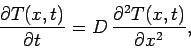(191)

for, subject to the mixed spatial boundary conditions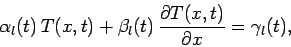(192)

at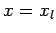, and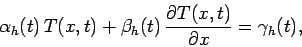(193)

at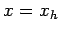. Here,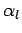,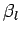, etc., are known functions of time. Of course,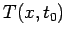must be specified at some initial time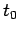.

Equation (191) needs to be discretized in both time and space. In time, we discretize on the equally spaced grid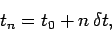(194)

where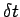is the time-step. Adopting a simple first-order differencing scheme, Eq. (191) becomes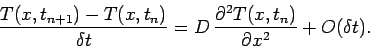(195)

In space, we discretize on the usual equally spaced grid-points specified in Eq. (114), and approximate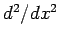via the second-order, central difference scheme introduced in Eq. (115). The spatial boundary conditions are discretized in a similar manner to Eqs. (134) and (135). Thus, Eq. (195) yields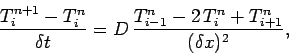(196)

or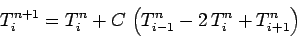(197)

for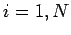, where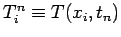, and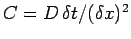. The discretized boundary conditions take the form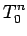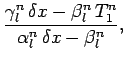(198)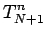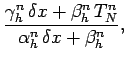(199)

where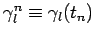, etc. The discretization scheme outlined above is termed first-order in time and second-order in space.

Equations (197)-(199) constitute a fairly straightforward iterative scheme which can be used to evolve the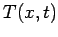in time.Next: An example 1-d diffusion Up: The diffusion equation Previous: Introduction
Richard Fitzpatrick 2006-03-29